# Sound Waves

Sound

Sound waves are longitudinal waves that travel through a medium. For longitudinal waves, the direction of motion of the particles is the same as the direction the wave travels. Sound can travel through many different mediums, though humans are most accustomed to hearing sounds through air. The human ear can hear sounds with frequencies between 20 and 20,000 Hz. This is called the human audible range. Sound waves begin at a source and travel outward in all directions. The amplitude of sound waves depends on the direction and distance from the source.

Pressure and Density

The term fluid can refer to either a liquid or a gas. When an object is immersed in a fluid, pressure is a perpendicular force exerted on an area of the object's surface,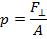The SI unit of force is the Newton (N), and the unit of area is meters squared (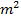). The unit of pressure is therefore Newtons per meter squared,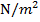. This unit is also known as the Pascal, for which the symbol is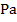.Density is the mass per unit volume of a material. Density is represented with the Greek letter("rho"). If a uniform material has mass m and volume V, then the density is,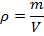The SI unit of density is kilograms per cubic meter,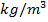. Grams per cubic centimeter is also commonly used. The conversion is,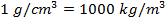Pressure and Sound Waves

Longitudinal waves can be thought of as pressure changes of the medium. Sound traveling through air consists of regions of compression and rarefaction, meaning regions of higher and lower pressure. This is equivalent to saying that these are regions of higher and lower density. For a sinusoidal wave in air, the pressure fluctuates around an equilibrium value. The ears of humans and animals are able to detect these pressure variations.

Sound waves traveling in one direction follow the wave formula from the previous section,In this formula,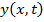is the position of a particle in the fluid, relative to its equilibrium position, along the x axis at a certain time t. A is the amplitude, k is the wave number, and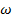is the angular velocity. When discussing sound waves, A is sometimes called the displacement amplitude. The wave number is related to the wavelength,As discussed in the previous section, the wavelength is also related to the frequency of a sound, by the formula for wave speed,For a certain medium, the wave speed is constant, so changes to the wavelength mean the opposite change to the frequency.

Perception of Sound

The loudness perceived by a listener depends on the wave number k (and therefore depends on the wavelength and frequency) and the amplitude of the wave. This means that the loudness depends on the frequency and amplitude of the sound.

The pitch of a sound depends on the frequency. High pitch sounds have a high frequency, and low pitch sounds have lower frequency. Another property of sound is tone or timbre, which depends on the harmonics that contribute to the sound (see the previous section). The timbre also depends on the shape of the wave function at the beginning and end of a tone. These are called the attack and decay of the tone.

Intensity of Sound

The intensity of a wave is the rate at which energy is transported by the wave, per unit area. The intensity of waves was first described in a previous section. Another way to define intensity is that it is the average power per unit area. Intensity is represented with the letter I, and the unit of intensity is Watts per square meter (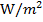).

For sound waves, the intensity is often expressed using the decibel scale. The decibel scale is logarithmic, meaning that it uses the mathematical function the logarithm to represent a large range of intensities in a smaller range of values. The Greek letter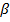("beta") is used to represent a sound intensity level on the decibel scale. The unit of the scale is the decibel, which is represented with the symbol dB. The definition of the unit is part of the definition of the scale itself. The formula for the sound intensity levelis,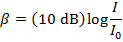In this formula, the intensity of a sound is divided by a reference intensity I0. The value of the reference intensity is,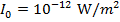If a sound has an intensity that is equal to the reference intensity,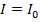, then on the decibel scale, the sound intensity levelis,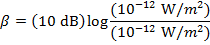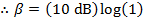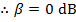Standing Waves

Sound waves inside a closed pipe reflect from the ends of the pipe. The original and reflected waves travel in opposite directions. These waves sum, according to the principle of superposition, and create certain places where the particles of the medium do not move. When this happens, a standing wave has been created. Sound waves are longitudinal waves, and so the particles move back and forth parallel to the direction of the wave. To distinguish this from the motion of transverse waves (see the previous section), the places in the wave pattern that are stationary, while the rest of the medium moves back and forth, are called displacement nodes. At the displacement nodes, the original and reflected waves cancel each other. Midway between the displacement nodes are positions where the particles move back and forth the largest distance. These are called the displacement antinodes. The distance between successive displacement nodes (or antinodes) is half of the wavelength,.

In a standing sound wave, the pressure and density at the displacement nodes varies significantly. These positions go through cycles of compression and rarefaction. In contrast, the displacement antinodes have constant pressure and density. For that reason, the displacement antinodes are also pressure nodes. They are positions where the pressure and density do not vary. The displacement nodes are therefore the pressure antinodes. To summarize,

Pressure nodes are always displacement antinodes, and pressure antinodes are always displacement nodes.

Normal Modes

Standing waves are the basis of the human voice and most musical instruments. When a standing wave is created inside a closed space, like a tube or pipe, it is possible to create normal modes. Normal modes of a sinusoidal wave occur when all of the particles of the medium move with the same frequency. The lowest frequency at which a normal mode is created is called the fundamental frequency. The higher frequencies at which normal modes are established are harmonics or harmonic frequencies, and these frequencies together form the harmonic series. These depend on the length of the pipe, and whether the pipe has a closed end, or is open at both ends.

When reflection takes place and the closed end of a pipe, the particles at this position cannot have any displacement. This means the closed end must be a displacement node, and therefore a pressure antinode. If the end of a pipe is open, it must be a pressure node. The open pipe is open to the atmosphere, and so at that position, the pressure cannot vary. This means the open end must be a pressure node, and therefore a displacement antinode.

An example of an instrument that uses open pipes is the organ. Organ pipes are open at both ends, and so the fundamental frequency must correspond to a standing wave pattern with a displacement antinode at each end, and a displacement node in the middle. If the length of the pipe is L, then the fundamental frequency f1 for a pipe open at both ends must be,(open pipe)

The normal mode frequencies for the pipe that is open at both ends are therefore,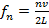(open pipe)

If the end of the pipe is closed, it is a stopped pipe. The fundamental frequency must correspond to a standing wave pattern with one displacement antinode (the open end), and one displacement node (the closed end). If the length of the pipe is L, then the fundamental frequency for a stopped pipe must be,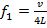(stopped pipe, closed at one end)

The normal mode frequencies must follow a different pattern, with odd values of n,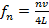(n=1,3,5, ...)  (stopped pipe, closed at one end)

Resonance

Mechanical resonance can occur when a varying force is applied to an oscillating system. The driving frequency is the frequency at which the force is applied, and the result is a forced oscillation. If the driving frequency is equal to one of the normal mode frequencies of the system, the amplitude of the oscillation can increase, and reach a maximum value much greater than the original oscillation amplitude.

Interference and Beats

When two or more waves overlap in the same region at the same time, the result is interference. The type of interference varies by position. Where the waves sum to produce a larger wave, the result is called constructive interference. Where the waves cancel to produce a smaller wave, or an amplitude of zero, the result is called destructive interference.

If two waves have the same amplitude, but slightly different frequencies, the constructive and destructive interference can vary with time. The result is called beats, which are variations of loudness. The frequency at which the combined sound reaches peak loudness is called the beat frequency. The beat frequency is the difference between the frequencies of the contributing sound waves. If fa is larger than fb, then the beat frequency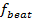is,The Doppler Effect

When the source of a sound and the listener are moving relative to each other, the frequency of the resulting sound is different than if they were at rest. This is known as the Doppler effect. To simplify the situation, assume that the source of the sound and the listener are moving either toward or away from each other.

In the following formula, the listener is labeled L, and the source is labeled S. The frequency at which the sound is emitted is fS. What is of interest is the difference between this and the frequency of the sound as heard by the listener, fL. The listener is moving at a velocity vL relative to the source. Assume that the positive direction is from the listener to the source, so a positive value of vL means that the listener is approaching the source of the sound, and a negative vL means the listener is moving away from the source. The source is moving at a velocity vS relative to the listener. A positive vS means that the source is moving away from the listener, and a negative vS means the source is moving toward the listener. The speed of sound in the medium is v. The frequency heard by the listener is,This formula means that, when the listener and source are moving closer, the frequency heard by the listener is higher than the source frequency. When the listener and source are moving farther away from each other, the frequency heard by the listener is lower than the source frequency.

 Related Links: Physics Quizzes AP Physics Notes Electric Charges